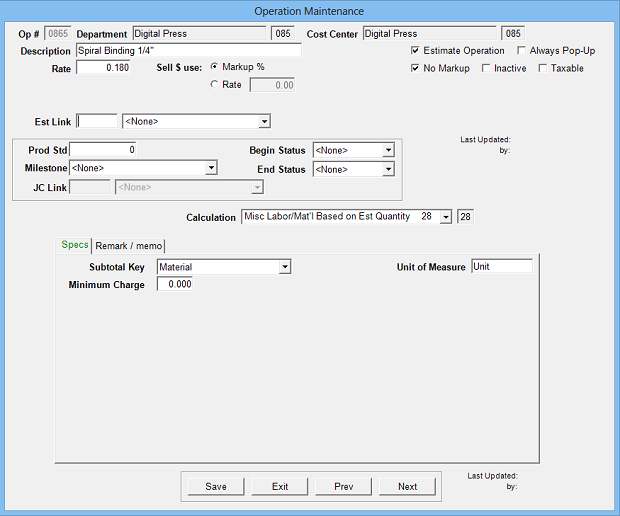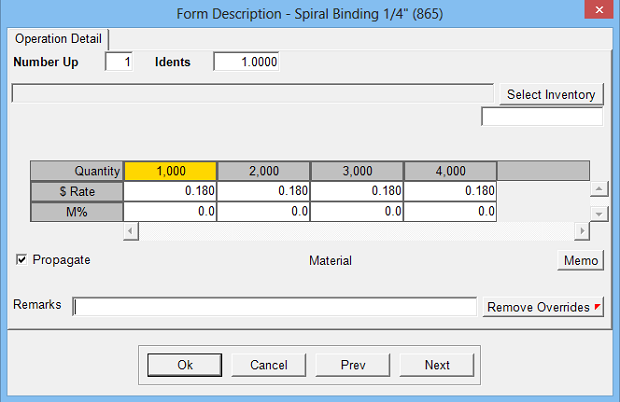Estimates the cost of labor, material or purchases where the number of units corresponds to the estimate quantity.

Operation Setup Requirements:
1. Description
2. Rate: Cost per unit
3. Sell \$ use: Markup % (recommended) or alternate sell rate
4. Calc: 28
5. Sub Key: Labor, Material or Fixed Purchase
6. Minimum Charge: Option to define minimum dollar chargeEstimate Specifications Input Prompts:

1. Number Up
2. Idents
3. Rate
4. J% or M%
5. Remarks
6. MemoCalculations:

(Estimate Quantity / Number Up) x Idents x Rate = Cost \$

If Cost \$ less than Minimum Charge, then Cost \$ = Minimum Charge

Cost \$ x Markup % = Markup \$

Cost \$ + Markup \$ = Sell \$